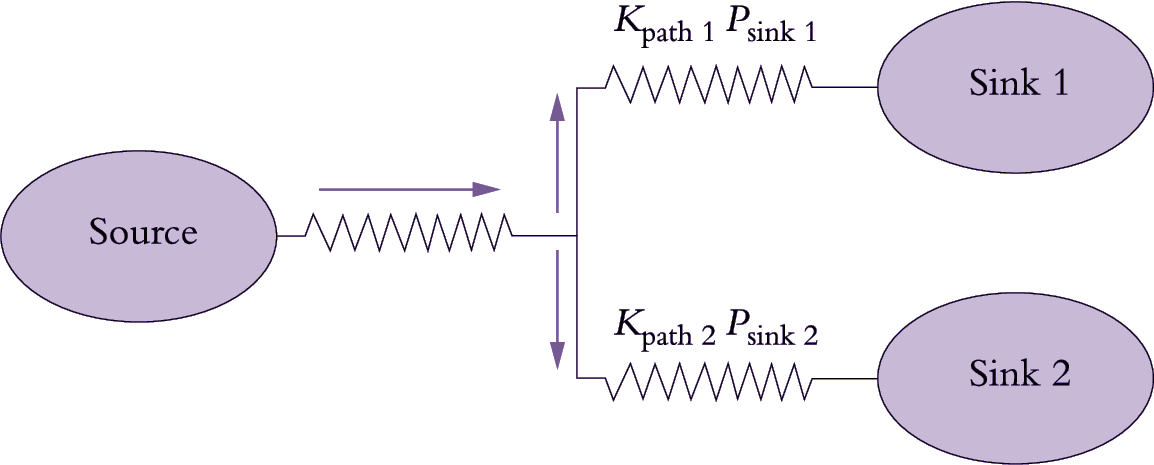# 5.2.5 - Control of assimilate transport from source to sink

Loading of sugars, potassium and accompanying anions into sieve tubes at sources determines solute concentrations in phloem sap (Table 5.1). The osmotic pressure (Π) of these solutes influences P generated in sieve tubes. Thus, source output determines the total amount of assimilate available for phloem transport as well as the pressure head driving transport along the phloem path to recipient sinks. Withdrawal of assimilates from sieve tubes at the sink end of the phloem path, by the combined activities of phloem unloading and metabolism/compartmentation (Table 5.1), determines Π of phloem sap. Other sink-located membrane transport processes influence Π around sieve tubes. The difference between intra- and extracellular Π of sieve tubes is a characteristic property of each sink and determines P in sink sieve tubes.

## Munns_PlantsInAction_Tab05.1.jpg.png.png

The pressure difference between source and sink ends of the phloem pathway drives sap flow (Equation 5.3) and hence phloem translocation rate (Equation 5.2) from source to sink. The source and sink processes governing the pressure dif-ference (Table 5.1) are metabolically dependent, thus rendering phloem translocation rates susceptible to cellular and environmental influences. The pressure-flow hypothesis predicts that the phloem path contribution to longitudinal transport is determined by the structural properties of sieve tubes (Table 5.1). Variables of particular importance are cross-sectional area (A) of the path (determined by numbers of sieve pores in a sieve plate and sieve-tube numbers) and radius of these pores (sets r in Equation 5.3). These quantities appear in Equations 5.2 and 5.3. Thus, the individual properties of each sink and those of the phloem path connecting that sink to its source will determine the potential rate of assimilate import to the sink (Figure 5.12).

## Fig_p_5.21.pngFigure 5.12. Scheme describing photoassimilate flow from a source leaf linked to two competing sinks, Sink 1 and Sink 2. Assimilate flows through alternative phloem paths (Path 1 and Path 2) each with its own conductance (Kpath) and pressure difference (P) between source and sink. Hence Path 1 is distinguished by Kpath1 and Psink1 and Path 2 by Kpath2and Psink2

The transport rate (R) of assimilate along each phloem path, linking a source with each respective sink, can be predicted from the pressure-flow hypothesis (see Equations 5.2 and 5.3) as:

$R = K_{path} (P_{source} - P_{sink}) C \tag{5.4}$

where path conductance (Kpath) is the product of path hydraulic conductivity (Lp) and cross-sectional area (A). Hence, the relative flows of assimilates between hypothetical sinks (sink 1 and sink 2) shown in Figure 5.12 may be expressed by the following ratio:

$\frac{K_{path1} (P_{source} - P_{sink1}) C} {K_{path2} (P_{source} - P_{sink2}) C} \tag {5.5}$

Partitioning of assimilates between two competing sinks is thus a function of path conductance and P at the sink end of the phloem path (Equation 5.5). Since phloem has spare capacity, any differences in the conductance of the inter-connecting paths (Figure 5.12) would exert little influence on the rate of phloem transport to the competing sinks. Assimilate partitioning between competing sinks would then be determined by the relative capacity of each sink to depress sieve-tube P at the sink end of the respective phloem path. Even when differences in path conductance are experi-mentally imposed, phloem transport rates are sustained by adjustments to the pressure differences between the source and sink ends of the phloem path (Wardlaw 1990).

These conclusions have led to a shift in focus from phloem transport to phloem loading and unloading, which are instrumental in determining the amount of assimilate translocated and its partitioning between competing sinks, respectively.